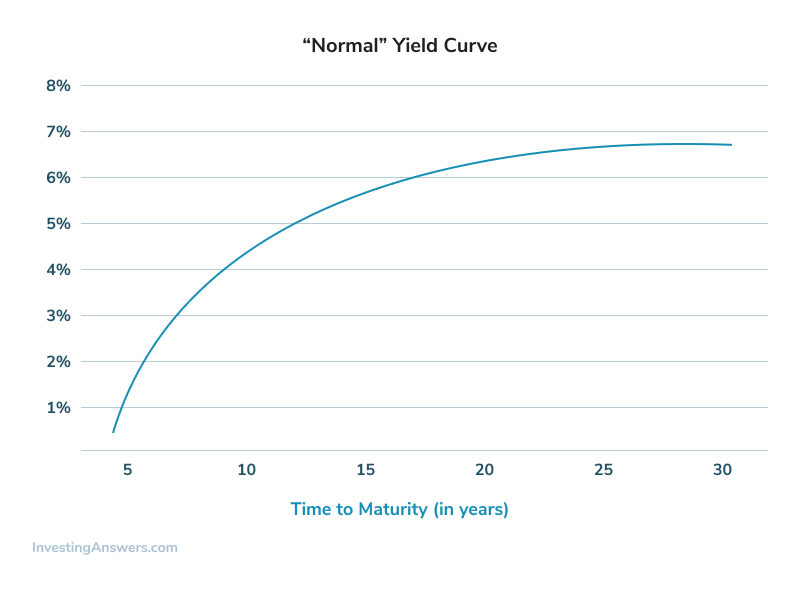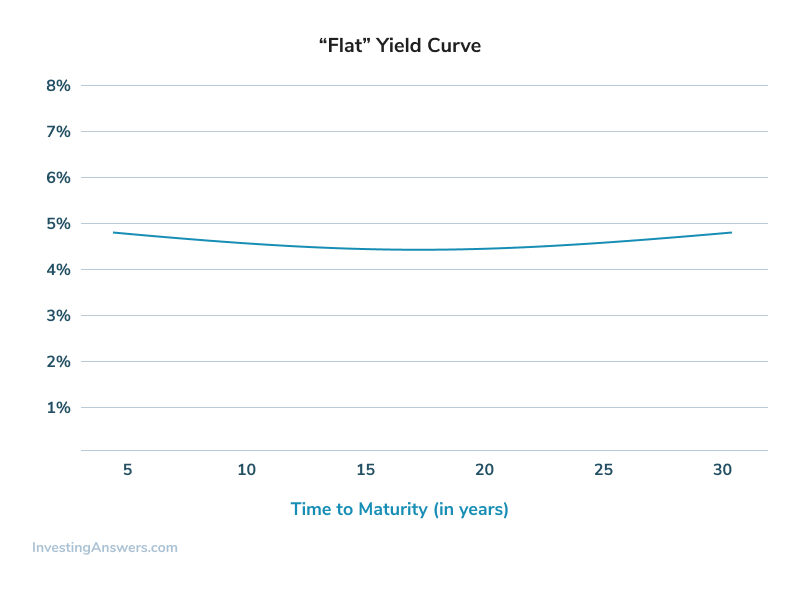## What is a Yield Curve?

The yield curve, also known as the 'term structure of interest rates,' is a graph that plots the yields of similar-quality bonds against their maturities, ranging from shortest to longest. (Note that the chart does not plot coupon rates against a range of maturities -- that's called a spot curve.)

## How Does a Yield Curve Work?

The yield curve shows the various yields that are currently being offered on bonds of different maturities. It enables investors at a quick glance to compare the yields offered by short-term, medium-term and long-term bonds.

The yield curve can take three primary shapes. If short-term yields are lower than long-term yields (the line is sloping upwards), then the curve is referred to a positive (or 'normal') yield curve. Below you'll find an example of a normal yield curve:If short-term yields are higher than long-term yields (the line is sloping downwards), then the curve is referred to as an inverted (or 'negative') yield curve. Below you'll find an example of an inverted yield curve:Finally, a flat yield curve exists when there is little or no difference between short- and long-term yields. Below you'll find an example of a flat yield curve:It is important that only bonds of similar risk are plotted on the same yield curve. The most common type of yield curve plots Treasury securities because they are considered risk-free and are thus a benchmark for determining the yield on other types of debt.

The shape of the yield curve changes over time. Investors who are able to predict how the yield curve will change can invest accordingly and take advantage of the corresponding change in bond prices.

Yield curves are calculated and published by The Wall Street Journal, the Federal Reserve, and a variety of other financial institutions.

## Why Does a Yield Curve Matter?

In general, when the yield curve is positive, this indicates that investors require a higher rate of return for taking the added risk of lending money for a longer period of time.

Many economists also believe that a steep positive curve indicates that investors expect strong future economic growth and higher future inflation (and thus higher interest rates), and that a sharply inverted yield curve means investors expect sluggish economic growth and lower inflation (and thus lower interest rates). A flat curve generally indicates that investors are unsure about future economic growth and inflation.

There are three main theories that attempt to explain why yield curves are shaped the way they are.

1. The 'expectations theory' states that expectations of rising short-term interest rates are what create a positive yield curve (and vice versa).

2. The 'liquidity preference hypothesis' states that investors always prefer the higher liquidity of short-term debt and therefore any deviance from a positive yield curve will only prove to be a temporary phenomenon.

3. The 'segmented market hypothesis' states that different investors confine themselves to certain maturity segments, making the yield curve a reflection of prevailing investment policies.

Because the yield curve is generally indicative of future interest rates, which are indicative of an economy's expansion or contraction, yield curves and changes in yield curves can convey a great deal of information. In the 1990s, Duke University professor Campbell Harvey found that inverted yield curves have preceded the last five U.S. recessions.

Changes in the shape of the yield curve can also have an impact on portfolio returns by making some bonds more or less valuable relative to other bonds. These concepts are part of what motivates analysts and investors to study yield curves carefully.